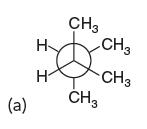Problem: Consider the following two conformations of 2,3-dimethylbutane. For each of these conformations, use Table 4.6 to determine the total energy cost associated with all torsional strain and steric strain.

88% (36 ratings)
Problem Details

Consider the following two conformations of 2,3-dimethylbutane. For each of these conformations, use Table 4.6 to determine the total energy cost associated with all torsional strain and steric strain.What scientific concept do you need to know in order to solve this problem?

Our tutors have indicated that to solve this problem you will need to apply the Barrier To Rotation concept. You can view video lessons to learn Barrier To Rotation Or if you need more Barrier To Rotation practice, you can also practice Barrier To Rotation practice problems .

What is the difficulty of this problem?

Our tutors rated the difficulty of Consider the following two conformations of 2,3-dimethylbuta... as high difficulty.

What professor is this problem relevant for?

Based on our data, we think this problem is relevant for Professor Duis' class at NAU.

What textbook is this problem found in?

Our data indicates that this problem or a close variation was asked in . You can also practice practice problems .